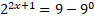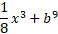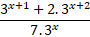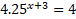# Mathematics Grade 10 - Exponents

Introduction

An exponent is an expression that consists of a repeating power of multiplication of the same element.

It is also known as indices or increased to the power of a number. Consider the formula 52, where the number 5 is the base and the number 2 is the exponent.

The value of the expression is obtained by multiplying the base by the number of powers. In the above example, the power is 2, hence the number is 5 x 5 = 25.

The assignment required of you is to answer the following QUESTIONS 1-4.

Each question is worth 5 marks and the total test is out of 20 marks.

Questions:

1)2)3)4)Process

Answer Each Question Chronologically.

Explain each Law used within each specific question.

Thereafter answer each question there.

Evaluation
Rubric
# 0-1 2-3 3-4 5
QUESTION 1
QUESTION 2
QUESTION 3
QUESTION 4

Conclusion

Therefore those are the basics within Exponents and the various types of exam type examples that one can expect within the examination process.

Credits

Credits :

Youtube.com. 2022. [online] Available at: <https://www.youtube.com/watch?v=-zUmvpkhvW8&gt; [Accessed 10 September 2022].

Teacher Page